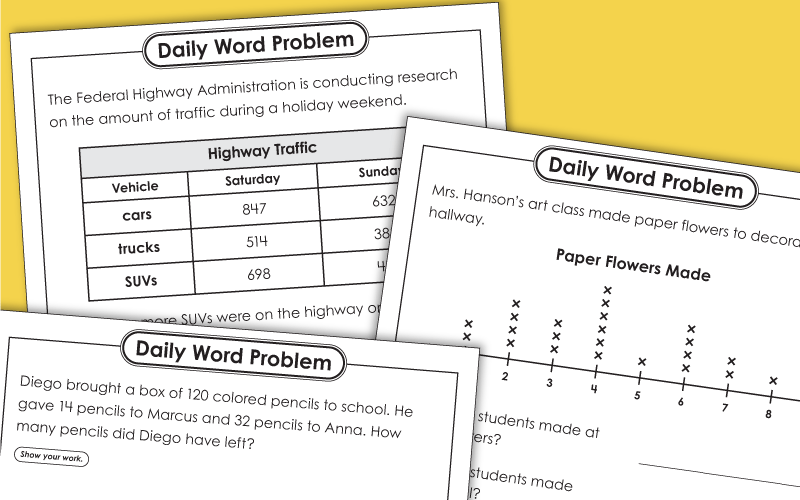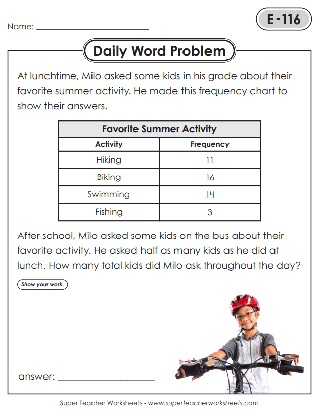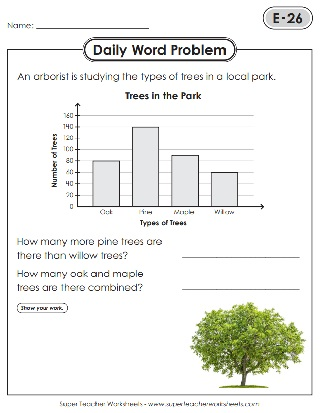# Daily Word Problems - Level E (5th Grade)

This page offers a wide variety of daily math word problems for a 5th grade level (Level E). Problems review a large range of skills, such as operations with fractions and decimals, place value, reading and interpreting graphs, number patterns, and much more.This file has 5 worksheets, one for each day of the week. Topics include adding with fractions, finding the area of a rectangle, and more.
Five worksheets are included in this file. Each worksheet features a daily math word problem. Topics include frequency charts, place value, and more.
These five worksheets reinforce key math skills for 5th grade. Each worksheet includes a daily word problem, most of which involve multiple steps. Topics include subtracting fractions, dividing money, and interpreting a chart.
Here are five more worksheets with daily word problems for 5th graders. Topics include finding volume, calculating time, and comparing decimals.
Skills used in these five worksheets include adding and subtracting decimals with money, finding the area of a rectangle, division with remainders, and more.
These worksheets reinforce key 5th grade math skills. Topics include reading and interpreting a bar graph and converting measurements.
These word problems reinforce key math skills, such as finding area and performing operations with decimals and fractions.
This file contains five daily worksheets with math problems for 5th graders. Skills include finding volume, adding fractions and decimals, and writing expressions.
This set of daily word problems features skills such as place value, division with remainders, and addition with decimals.
These worksheets cover topics such as rounding decimals to the nearest whole number, converting improper fractions to mixed numbers, and more.
Skills covered in these daily worksheets include reading and interpreting frequency charts, reducing fractions, and adding fractions with unlike denominators.
Each worksheet contains a daily word problem which reinforces key math skills. This set requires students to perform operations with decimals and fractions.
These daily word problems deal with skills such as measurement, operations with fractions with unlike denominators, and operations with decimals.
This set of daily word problems includes a tape diagram, multiplying fractions, measurement conversions, and more!
This group of word problems includes calculating area with fractions, recognizing and following patterns, and more 5th-grade math skills.
The daily word problems in this file feature questions involving adding and subtracting money, division with remainders, and interpreting fractions
This set of 5th grade daily word problems features a line plot, units of measurement conversions, and multiplying fractions.
The next five daily word problems require students to use skills such as reading and interpreting a table, and performing operations with decimals and fractions.
These multistep problems reinforce skills such as finding the area of a rectangle, writing equations, and performing operations with fractions and decimals.
With this group of daily word problems, students will practice writing equations, ordering decimals, multiplying fractions, and more.
The next set of daily word problems includes calculating the area of a rectangle with mixed numbers, multiplying with decimals and fractions, and more.
This set of daily word problems includes calculating volume, comparing fractions, and more key elementary math concepts.
With this set of daily word problems, students will practice adding and multiplying fractions and mixed numbers, interpreting a chart, and performing operations with decimals.
With this set of daily word problems, students will calculate the length of a rectangle using its perimeter and width, read and interpret a frequency chart, find the volume of a pool, and practice more math skills.
With these worksheets, students will convert teaspoons to cups and cups to gallons, perform equations with mixed numbers or decimals, and more.
Practice a variety of math skills, such as dividing with decimals, with this next set of daily word problems.
This set of daily word problems reinforces key math skills, including reading and interpreting a pay stub.
These daily word problems help students practice important 5th grade math skills, such as calculating additive volume.
With these worksheets, students will practice multiplying fractions, dividing decimals, and much more.
Practice calculating volume, converting pounds to ounces, and more with this last set of 5th grade daily word problems.
Math Buzz

Our Math Buzz set includes all sorts of daily math practice problems, from place value to operations with fractions and decimals.

More Daily Word Problems

Check out our Daily Word Problems for other grade levels!

## Sample Worksheet ImagesMy Account
Site Information